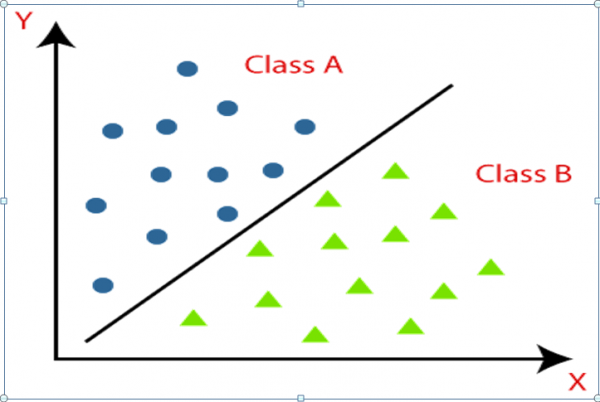# MACHINE LEARNING:- Classification part I

Posted

This article focus on introducing ML and its use in classification. Mathematical principles have not been covered in it. We will cover some mathematical details and also the basics algorithms in next few articles.

1

### MACHINE LEARNING:- Classification, A General Intuition

Well, we have been listening to a lot about machine learning aka ML nowadays. But what is machine learning and why it is so popular? Well, I know you are all curious and so am I, let’s start exploring this field. we will first see what machine learning is and then overview one of its use in the classification of objects. So let’s get started (:

So what is machine learning? Is it teaching some machine? Well yes but no. It is way more.

Machine Learning is the science of getting a machine to learn and act like humans do, and improve their learning over time in an autonomous fashion, by feeding them data and information in the form of observations and real-world interactions

Well, that sounds too complex so in short ML is about teaching a machine to perform the different tasks by giving them data and training them to adapt their thinking according to that data.

For example, when you were a child, your parent teaches you how to speak by speaking different words. Then you listen to those words and start learning or I can say adapting to those words. So basically here you are machine, words are data and your parent was the programmer, which means yeah they were(:

So now you will ask me what these ml do? ML does many things from predicting the price of a stock to running a car without a driver. But right now, we are not into these instead we want to learn one of the most important applications of machine learning and that is classification. Now you will ask what is classification? I know you know its meaning but what is classification in sense of machine learning. So let us find out.

In machine learning, classification refers to a predictive modeling problem where a class label is predicted for a given example of input data. Again it sounds too complex so let me make it easy. Classification is a method to differentiate between different objects by observing different features with the help of examples aka data. A classification can be a binary mean classification of two object types or it can be multiple mean classifications of more than two objects type.

So now you know about ML and also about classification so should we stop. Of course not let us dig deep into classification. SO how can a machine classify, is it some kind of magic or there is some logic behind it. Every tool of ML is based on the simple principle of mathematics, especially statistics. We use different principles like likelihood, Gaussian theorem to make our classification. So now how to know about all these mathematical concepts. Don’t worry I will explain every mathematical principle in upcoming notes or curious to start exploring now.

So again coming back to classification, let’s talk about binary classification. Let’s talk about the cat VS dog example.  So we want to create a classification model( classifier) to classify between dog and cat. So for that first, we need some data about cats and dogs. Our classification depends upon the no of data points we have. In our dataset, we will have different features like weight, eye color, height, etc. When we feed this dataset to our classifier it would apply some mathematical modeling and will start learning from that example. It would add different weights to different features in our dataset and will create a classification boundary between the cat and dog. When we would feed an unknown data point to our classifier it would set the value of coefficient on that point and accordingly classify it as cat or dog.So from the above graph, our class A would-be cat, and B would be a dog. The x and y-axis represent different feature and the line would represent our classification boundary aka it divide our data points into two classes. This is only a simple example without talking about mathematics and the programming behind it. But in coming notes we will talk in-depth about classification so just hang on to know more(:

Note: This is just an introduction to develop intuition about classification. If you don’t understand something write back and please ignore any grammatical mistakes.